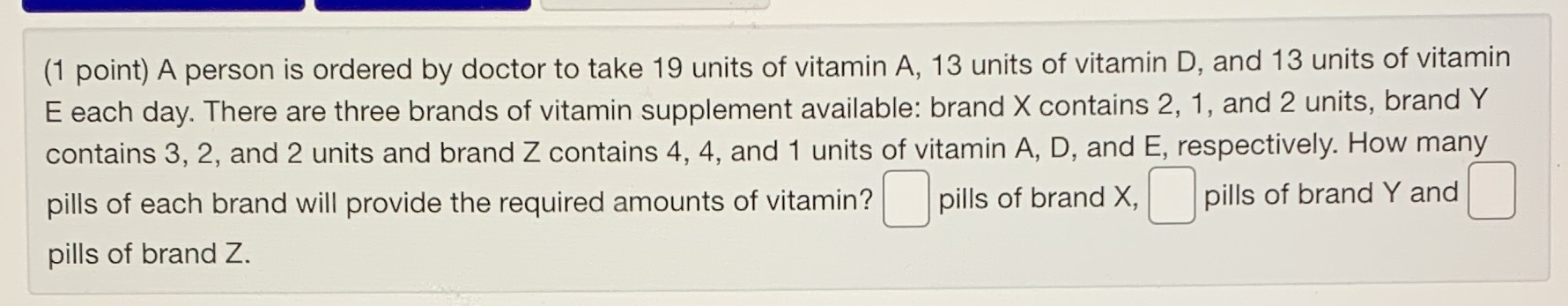### Still have math questions?$$1$$ point) A person is ordered by doctor to take $$19$$ units of vitamin A, $$13$$ units of vitamin D, and $$13$$ units of vitamin E each day. There are three brands of vitamin supplement available: brand X contains 2, 1, and $$2$$ units, brand Y contains 3, 2, and $$2$$ units and brand Z contains 4, 4, and $$1$$ units of vitamin A, D, and E, respectively. How many pills of each brand will provide the required amounts of vitamin? pills of brand Z.
$$3,3,1$$